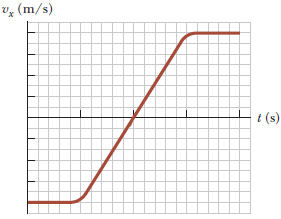Jeremy A.A box proceeds along the x-axis and the figure below shows a record of its velocity as a function of time. Every gridline along the vertical axis corresponds to 2.00 m/s and each gridline along the horizontal axis corresponds to 0.500 s. (Enter your answers in m/s2. Indicate the direction with the signs of your answers. Note that t = 0

at the intersection of the axes.)

(a) Determine the average acceleration of the box in the time interval t = 0

to t = 2.50 s

(b) Determine the average acceleration of the box in the time interval t = 2.50 s

to t = 7.50 s.

(c) Determine the average acceleration of the box in the time interval t = 0

to t = 10.0 s.

By:Tutor
5 (20)

Math Tutor with Experience

## Still looking for help? Get the right answer, fast.

Get a free answer to a quick problem.
Most questions answered within 4 hours.

#### OR

Choose an expert and meet online. No packages or subscriptions, pay only for the time you need.